# Distance between Two Points

Distance between Two Points
Go back to  'Coordinate Geometry'

 1 Definition of Distance between Two Points 2 Formula for Distance between Two Points 3 Proof of Distance Formula 4 Important Notes on Distance between Two Points 5 Solved Examples for Distance between Two Points 6 Distance Between Two Points Calculator 7 Practice Questions for Distance between Two Points 8 Challenging Questions on Distance Formula 9 Maths Olympiad Sample Papers 10 Frequently Asked Questions (FAQs) 11 FREE Worksheets on Distance between Two Points

We at Cuemath believe that Math is a life skill. Our Math Experts focus on the “Why” behind the “What.” Students can explore from a huge range of interactive worksheets, visuals, simulations, practice tests, and more to understand a concept in depth.

Book a FREE trial class today! and experience Cuemath's LIVE Online Class with your child.

## Definition of Distance between Two Points

The distance between any two points is the length of the line segment joining the points.

For example, if $$A$$ and $$B$$ are two points and if $$\overline{AB}=10$$ cm, it means that the distance between $$A$$ and $$B$$ is $$10$$ cm.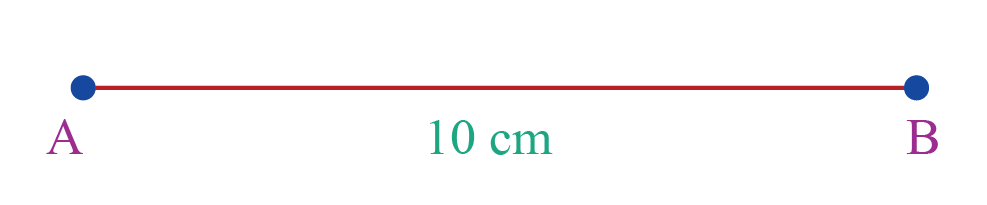The distance between two points is the length of the line segment joining them (but this CANNOT be the length of the curve joining them).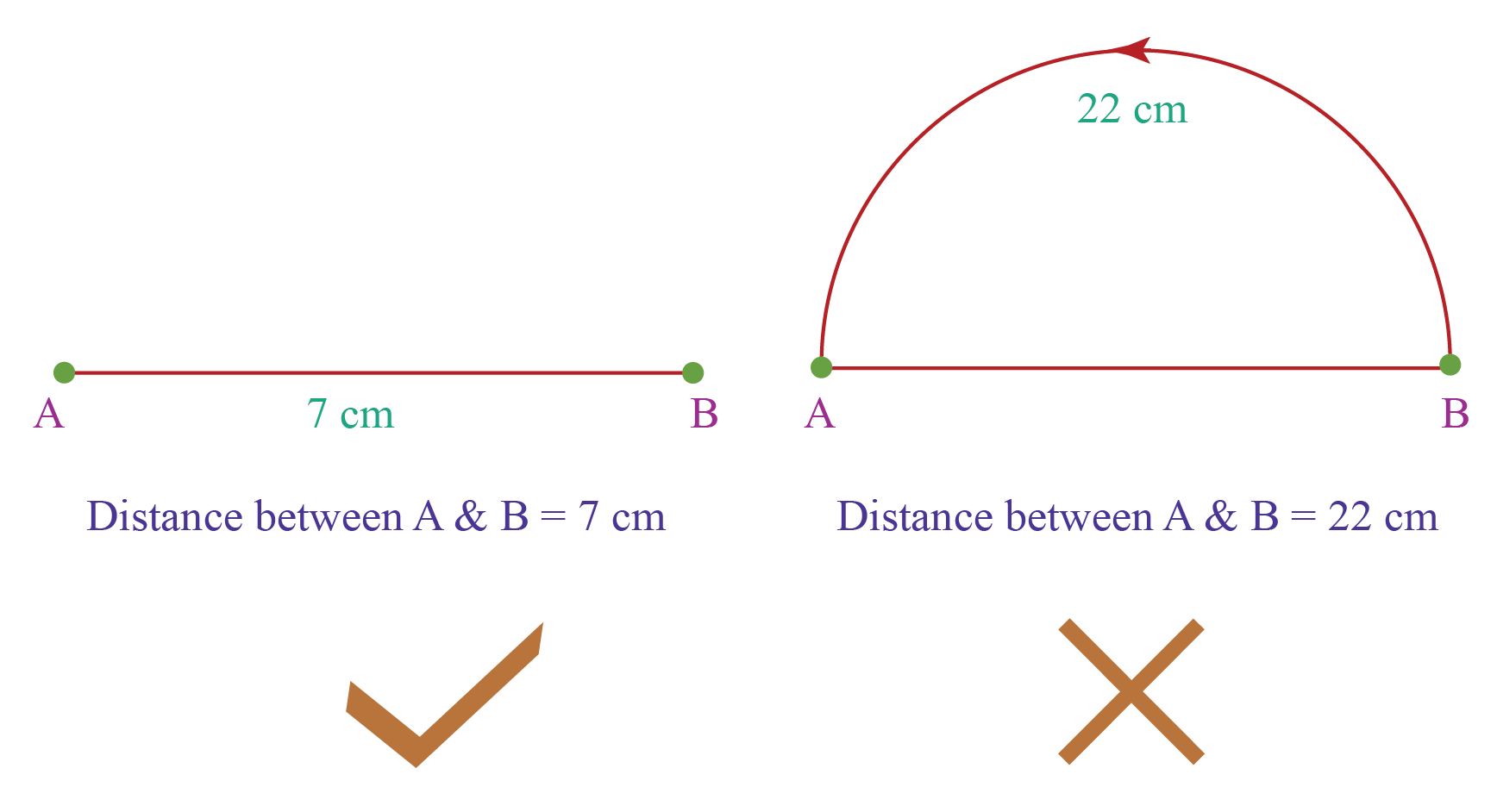How do we find the distance between two points if their coordinates are given?

## Formula for Distance Between Two Points

The formula for the distance, $$d$$, between two points whose coordinates are $$(x_1, y_1)$$ and $$(x_2, y_2$$) is:

 $d = \sqrt{(x_2-x_1)^2+(y_2-y_1)^2}$

This is called the Distance Formula.

Let's learn how to derive this formula next.

## Proof of Distance Formula

Let us assume that:

$A = (x_1, y_1)\\[0.2cm]B=(x_2, y_2)$

Next, we will assume that $$\overline{AB}=d$$

Now, we will plot the given points on the coordinate plane and join them by a line.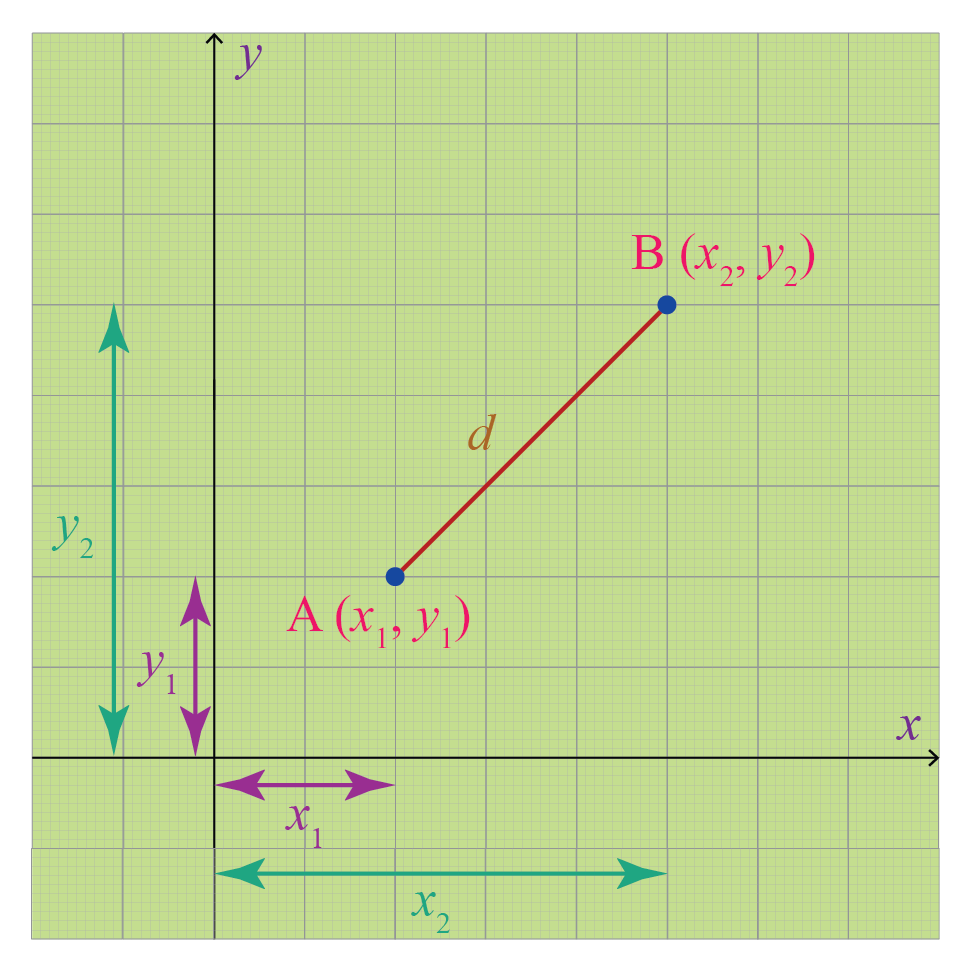Next, we will construct a right-angled triangle with $$\overline{AB}$$ as the hypotenuse.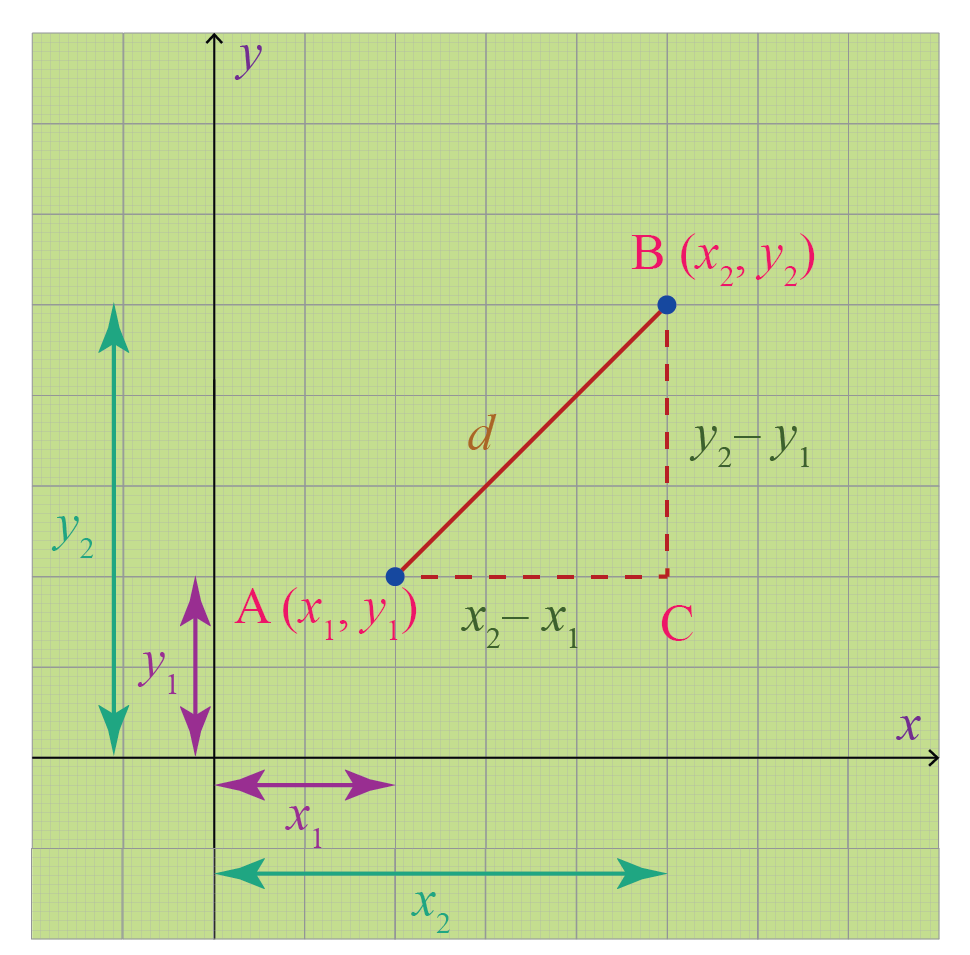Applying Pythagoras theorem for the $$\triangle ABC$$:

\begin{aligned} AB^2 &\!=\! AC^2\!+\!BC^2\\d^2 &\!=\! (x_2\!-\!x_1)^2\!+\!(y_2\!-\!y_1)^2 \,\, \text{[Values from the figure] }\\d &\!=\! \sqrt{(x_2\!-\!x_1)^2\!+\!(y_2\!-\!y_1)^2} \, [ \text{Taking square root on both sides} ] \end{aligned}

Thus, the distance formula is proved.

Help your child score higher with Cuemath’s proprietary FREE Diagnostic Test. Get access to detailed reports, customized learning plans, and a FREE counseling session. Attempt the test now.

## Solved Examples

 Example 1

Find the distance between the two points $$(2, -6$$) and $$(7, 3$$)

Solution:

Let us assume the given points to be:

\begin{aligned} (x_1,y_1)&=(2, -6)\\[0.2cm](x_2, y_2)&= (7,3) \end{aligned}

The formula to find the distance between two points is:

\begin{aligned} d &=\sqrt{(x_2-x_1)^2+(y_2-y_1)^2}\\[0.3cm] d &= \sqrt{(7-2)^2+(3-(-6))^2}\\[0.3cm] d&= \sqrt{5^2 + 9^2}\\[0.3cm] d&= \sqrt{25+81}\\[0.3cm] d &= \sqrt{106} \end{aligned}

 $$\therefore$$ Distance $$= \sqrt{106}$$
 Example 2

Show that the points $$(2, -1), (0, 1)$$ and $$(2, 3$$) are the vertices of a right-angled triangle.

Solution:

Let us assume the given points to be:

\begin{aligned} A &=(2,-1)\\[0.2cm] B&=(0,1)\\[0.2cm] C&=(2,3)\\[0.2cm] \end{aligned}

We will find the distance between every two points using the distance formula.

\begin{aligned} AB&\! =\! \sqrt{(0-2)^2+(1-(-1)^2}\!=\! \sqrt{(-2)^2+(2)^2}\!=\! \sqrt{4+4}\!=\! \sqrt{8}\\ BC &\!=\! \sqrt{(2-0)^2+(3-1)^2}\!=\! \sqrt{(2)^2+(2)^2}\!=\! \sqrt{4+4}\!=\! \sqrt{8}\\ CA &\!=\! \sqrt{(2-2)^2+(3-(-1))^2}\! =\! \sqrt{0^2+4^2}\!=\! \sqrt{16}\!=\!4\end{aligned}

Now that we know the lengths of all three sides,

\begin{aligned} AB^2+BC^2 &= CA^2 \\[0.3cm] (\sqrt{8})^2 +(\sqrt{8})^2 &= 4^2 \\[0.3cm] 8+8&=16\\[0.3cm] 16&=16 \end{aligned}

Thus, $$A, B$$ and $$C$$ satisfy the Pythagoras theorem.

So $$\triangle ABC$$ is a right-angled triangle.

We can prove the same by marking all the coordinates on a graph: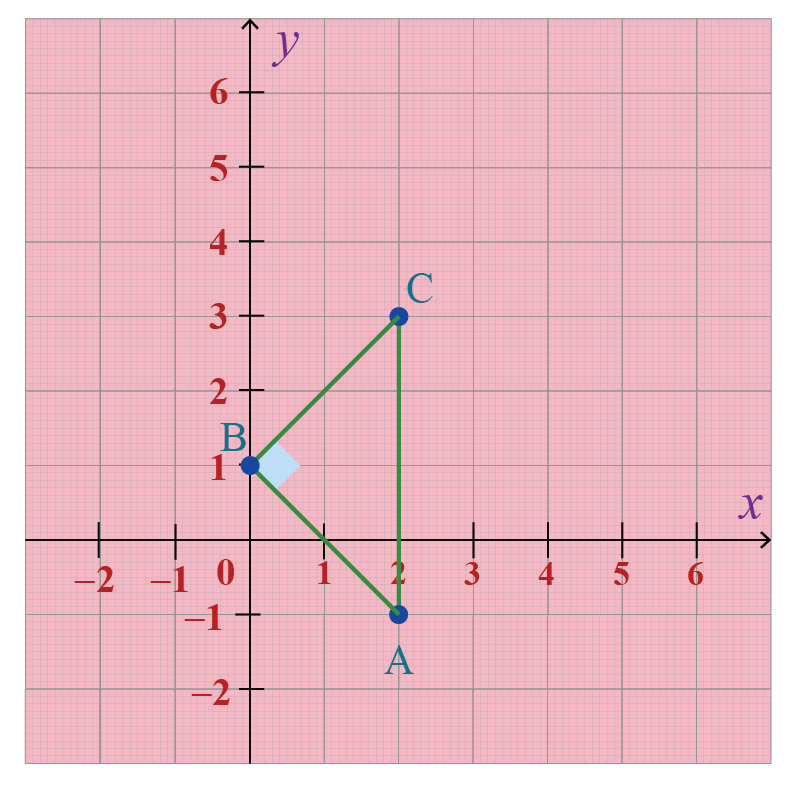Thus,

 The given points form a right-angled triangle.
 Example 3

Find a point on the y-axis that is equidistant from the points $$(-1, 2$$) and $$(2, 3)$$

Solution:

We know that the x-coordinate of any point on the y-axis is $$0$$

Hence, we assume the point that is equidistant from the given points to be $$(0, k$$). i.e.,

Distance between $$(0, k)$$ and $$(-1, 2)$$ $$=$$ Distance between $$(0, k)$$ and $$(2, 3)$$

\begin{aligned} \sqrt{(-1-0)^2+(2-k)^2}&= \sqrt{(2-0)^2+(3-k)^2}\\ \end{aligned}

Squaring on both sides

\begin{aligned}(-1-0)^2+(2-k)^2 &=(2-0)^2+(3-k)^2 \\1 + 4+k^2-4k &= 4+ 9 +k^2-6k \\2k &=8 \\ k&=4 \end{aligned}

Therefore, the required point is, $$(0, k)=(0, 4)$$

 $$\therefore$$ Required Point  $$= (0, 4)$$

## Distance between Two Points Calculator

Here is the "Distance Between Two Points Calculator".

Here, you can enter the coordinates of two points and it will show you the distance between them with the step-by-step explanation.

CLUEless in Math? Check out how CUEMATH Teachers will explain Distance between Two Points to your kid using interactive simulations & worksheets so they never have to memorise anything in Math again!

Explore Cuemath Live, Interactive & Personalised Online Classes to make your kid a Math Expert. Book a FREE trial class today!

## Practice QuestionsChallenging Questions
1. The vertices of a rectangle are $$(-4, -3), (4, -3), (4, 3),$$ and $$(-4, 3)$$. What is its area?
2. The vertices of a right-angled triangle are $$(-3, 6), (1, 6)$$ and $$(1, -1)$$. What is its area?

IMO (International Maths Olympiad) is a competitive exam in Mathematics conducted annually for school students. It encourages children to develop their math solving skills from a competition perspective.

## 1. What is the definition of the distance between two points?

The distance between any two points is the length of the line segment joining the points.

For example, if $$A$$ and $$B$$ are two points and if $$\overline{AB}=10$$ cm, it means that the distance between $$A$$ and $$B$$ is $$10$$ cm.## 2. What is the formula for the distance between two points?

The distance, $$d$$, between two points whose coordinates are $$(x_1, y_1)$$ and $$(x_2, y_2$$) is:

 $d = \sqrt{(x_2-x_1)^2+(y_2-y_1)^2}$

This is called the distance formula.

Coordinate Geometry
Grade 9 | Questions Set 1
Coordinate Geometry